# How to Optimize the Frequency Response of Buck Regulator ICS Externally

### Abstract

This application note describes how external compensation works and provides a method to implement it with above-mentioned devices. Through external compensation, the frequency response of buck switching regulators is adjusted to increase or decrease the bandwidth of the system by addition of a simple RC network with a feedback circuit.

### Introduction

Most Analog Devices switching regulators allow the user to adjust the internal control loop. This is typically achieved by varying PGM (programming) components to select alternative configurations from a selection of finite possible configurations. However, if the user requires greater flexibility, then external compensation is implemented. This application note provides the guidelines on the implementation of external compensation for the buck switching regulators that use CMC to optimize its frequency response i.e., increasing or decreasing the bandwidth of the system.

### Theoretical Overview

#### Why are Control Loop Adjustments needed?

There are several reasons as to why the adjustment of the control loop is needed. Mainly, adjustments are required to resolve an unstable loop. An unstable loop can reduce the performance of a device. This can include the unreasonable oscillation in the output voltage or excessive jittering of the switching waveform.

Alternatively, adjustments to the control loop may be desired by the user to increase the bandwidth of a device, which improves the transient performance. Adjustments may also be required if the user wishes to reduce the output capacitance to reduce BOM but still maintain a stable loop.

Figure 1 shows the approximate gain magnitude response of a typical buck converter that uses CMC.As shown in Figure 1, the typical CMC buck converter frequency response includes an intrinsic pole and zero at ωO and ωESR respectively. The pole ωO is the 'output load pole'. Its location is proportional to the load current applied. And the zero ωESR is generated by the equivalent series resistance (ESR) of output capacitors. Typically, this zero is either canceled by a pole in the device's internal compensation or pushed to a high enough frequency such to make its effects negligible.

Generally, the CMC systems are internally compensated using Type II compensation (PI) which improves the steady state error and provides flexibility in crossover frequency. Figure 2 shows the magnitude response of typical CMC with Type II compensation (Near crossover).Typical Buck converter gain magnitude response (CMC) with type II compensation

It is possible that the intrinsic pole and zero, alongside the internal compensation in place, do not give the user's required frequency response. In this case, the user can begin by adjusting the internal control loop of the IC, harnessing the programmability offered by the specific part. An external compensation is needed if this does not meet the user's requirements. This is realized in the form of lead or lag compensation by adding RCs (a resistor and capacitor in series) in parallel to the feedback resistors.

The objective of lead compensation is to introduce a zero and a pole at frequencies fZ and fP respectively, where fZ < fP. The following are the possible motivations to introduce the lead compensation:

• Higher Bandwidth (and therefore reduce rise time and settling time)
• Faster transient response
• Improved Stability (via an increase in Phase Margin)

A possible drawback of lead compensation is that it may increase high-frequency noise. To prevent this, a pole is placed at higher frequency.

Figure 3 shows the effect that lead compensation has on magnitude and phase. In this example, fZ = 10Hz and fP = 10kHz is used.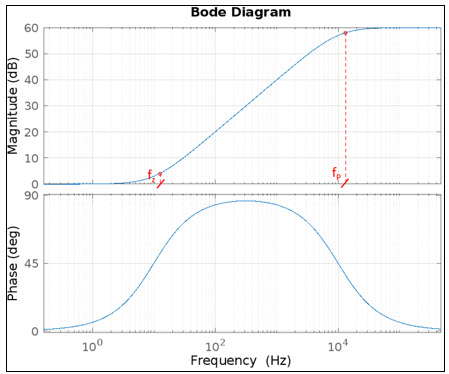#### Lag Compensation

The objective of lag compensation is to introduce a pole and a zero at frequencies fP and fZ respectively, where fP < fZ (<< BW). The key motivations for using lag compensation are to reduce bandwidth or to improve the steady-state response and have a low DC error.

Figure 4 shows the effect that lag compensation has on magnitude and phase. In this example, fP = 10Hz and fZ = 10kHz is used.#### Control Loop Bandwidth (BW) for CMC Buck Converters

For point-of-load buck converters (MAX20710, MAX20812, etc.), the control loop bandwidth is proportional to the feedback divider ratio and calculated by the following equation:Equation 1

Where,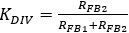is the Feedback Divider from VOUT to VSENSE

COUTis the Output Capacitance

G is the programmable loop gain (Obtained from the IC data sheet)

For a given "G "and "COUT" (Fixed based on circuit requirements), the BW reduces to:Equation 2

Therefore, by varying the feedback ratio while maintaining its DC ratio constant (to maintain the required reference voltage), the user can increase or decrease the Bandwidth.

* COUT is calculated including its derating at rated output voltage

* This method of external compensation is valid for all ICs with BW proportional to feedback ratio (Follows Equation 2)

### Circuit Implementation

Lead and lag compensation is implemented by adding RC network (a resistor and capacitor in series) in parallel to the feedback resistors, which are external to the switching regulator IC. For lead compensation (as shown in Figure 5a), an RC is added in parallel to the top feedback resistor (RFB1). While for lag compensation (as shown in Figure 5b), an RC is added in parallel to the bottom feedback resistor (RFB2).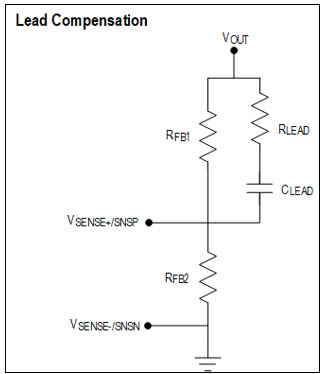#### Effect of Compensation on Loop Gain - T(s)

For a buck regulator, a typical loop gain T(s) is shown in Figure 6.The loop gain T(s) is written as:

T(s) = GC(s) × GVC(s) × H(S)

Where,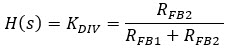From Equation 2

Where,
GC(s) is internal compensator TF
GVC(s) is control to output TF of the buck switching regulator

With lead compensation included, the term H(s) gets modified to the following: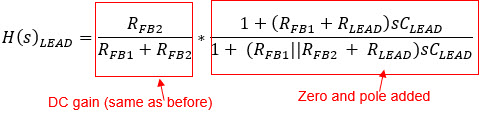Equation 3

The series combination of RLEAD and CLEAD adds a zero and pole at fZ and fP given by: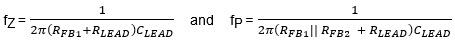Result 1

And it is observed that FZ < FP

Thereby, updated loop gain T(s)LEAD is: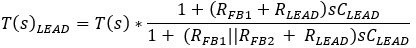Equation 4

Equation 4 is further analyzed in detail in the next section.

2. Lag Compensation:

Like lead, with lag compensation included, the term H(s) gets modified to:Equation 5

The series combination of RLAG and CLAG adds a zero and pole at fZ and fP given by:Result 2

And it is observed that fP < fZ

Thereby with lag compensation, the loop gain is:Equation 6

Equation 3 and Equation 5 confirms that, H(s) = KDIV (i.e., directly proportional to BW as per Equation 2), gets modified based on the pole-zero pair's location. The added pole-zero pair provides certain gain/attenuation based on the frequency and the values of RLEAD/LAG and CLEAD/LAG. This is used to either increase or decrease the current BW.

Hence, adding a series RC network across the feedback resistors modified the system loop gain, which ultimately modified the effective BW of the converter. To analyze the effect of newly added pole and zero, lead and lag compensation are considered separately. Also, the gain provided by the pole-zero pair is dependent on frequency, hence the frequency domain approach of Bode plot is used to analyze the results obtained.

#### Analysis of Loop Gain with Lead Compensation

The main objective of lead compensation is to obtain the maximum improvement in BW for the loop gain T(s). This section provides a design method for component selection (RLEAD and CLEAD) with goal of obtaining the maximum BW for a given system.

Figure 7 shows a typical compensated current mode control system. With lead compensation (externally) included, following is the response(shows typical response near the system crossover shown in Figure 7a):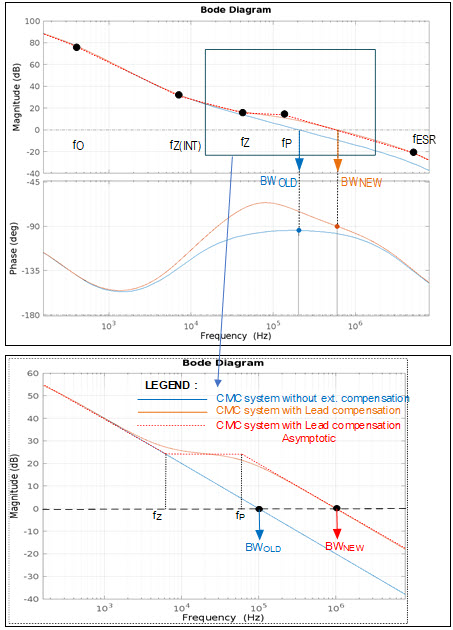It is observed that the newly added zero and pole at fZ and fP causes the system with lead compensation to have additional gain starting from fZ, this effectively causes the BW to improve as the compensated system crosses the 0dB line at BWNEW.

From the plot shown in Figure 4, it is concluded through geometry that:

Log(ƒp) - Log(ƒz) = Log(BWNEW) - Log(BWOLD)

Which implies,

BWNEW = BWOLD × (ƒp / ƒZ) Equation 7

Here,
BWNEW - Represents the new crossover frequency with lead compensation
BWOLD - Represents the crossover frequency with no external compensation

Equation 7 is an important conclusion as it directly links the BW improvement to the ratio of pole-zero pair frequency. This equation is used to derive values of RLEAD and CLEAD which provide the maximum bandwidth improvement.

Also, from Equation 7, it is clear that, for maximum BW, the pole-zero frequency ratio should be maximum.

Mathematically (substituting Result 1 into LHS of Equation 7), the ratio is simplified as:Equation 8

From analysis, it is observed that the maximum value of Equation 8 is obtained when:

Since the recommended values of RFB1, RFB2 are in between 1kΩ to 5kΩ, the user can choose RLEAD to be any value <5Ω.

Therefore, for the given values of feedback resistances, the BWNEW is calculated as per the Equation 7a: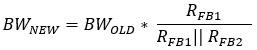Equation 7a is a useful tool to calculate the maximum BWNEW that is achieved for a given feedback resistance values (i.e., for a given voltage).

With the BW maximized thru careful selection of RLEAD, the value of CLEAD decides the location of fZ and fP. The location of the pole-zero pair also affects the Bandwidth and Phase Margin improvement.

To obtain the best possible case, the pole-zero location are swept from low frequency (as low as 100Hz) to the highest frequency possible (determined by BWOLD) by keeping the RLEAD constant and varying the CLEAD value.

Here, the highest frequency is the one that provides the least value of CLEAD, it is obtained when fZ = BWOLD. Choosing a frequency higher than this provides no lead compensation for the system. To avoid this, choose CLEAD greater than the following:Thorough simulation and hardware testing on multiple system configurations yielded the following results:

• Placement of the pole-zero pair near the highest frequency (before BWOLD) does not provide the maximum improvement in BW as the magnitude plot (actual plot) crosses over much earlier compared to the asymptotic plot. But this method provides a maximum increment in phase margin for the system.
• Placement of the pole-zero pair at lower frequencies (< 1kHz) results in a drastic reduction of PM for the system but also provides the maximum improvement in BW.

Therefore, the optimal location of the pole-zero pair is where the BW is maximum, and the PM reduction is minimum. That is found somewhere in-between the high and low frequencies.

Hence, for the maximum improvement in BW with slight reduction in PM, Equation 9 is used to calculate the value of CLEAD. This result is obtained by placing fP at 1/10th of BWOLD as shown below: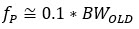This implies, CLEAD is calculated as:Equation 9

For example, if the requirement of the system is not just maximum BW but improvement in both BW and PM, then the CLEAD is decreased to adjust for the best case required. Decreasing the CLEAD reduces the BW improvement but increases the PM of the system.

The following are the ranges of CLEAD:

1. For improvement in both BW and PM but not max BW: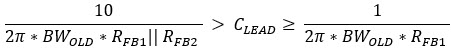2. For maximum BW (with no improvement in PM):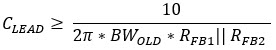3. For case 2, if the CLEAD increases, the PM of the system decreases.

#### Lead Compensation Hardware Testing and Results

The above theory is tested using the MAX20710 and MAX20812 EV kits. Multiple COUT values were used to verify the concurrence of the theoretical and practical results. The result of one such is shown here.

For the test, a series R-C network is added in parallel with RFB1 for lead compensation and values of the series element calculated using the equations mentioned above. The components used for the experiment have tolerance of 1% (RLEAD and CLEAD).

The test conducted uses the Equation 9 to calculate CLEAD. The main aim for the test is to obtain maximum BW with minimum decrease in PM.

MAX20710 EV kit results with COUT = 1600µF

Following are the values used for the test, it also includes the fZ and fP values obtained with the components used.

 RFB1 and RFB2 VOUT RLEAD (Ω) CLEAD (Calculated) CLEAD (Used in Ckt) fZ (kHz) fP (kHz) 1.87kΩ and 3.48kΩ 1V 0 19.5nF 18.3nF 4.67 7.21
 - BW (kHz) PM (°) No Load Uncompensated 67.436 58.876 Lead compensated 98.408 49.769 Full Load Uncompensated 69.285 58.037 Lead compensated 112.604 50.257

According to Equation 7a, the maximum improvement achievable is 103.673kHz.

From the results of Table 2, it is observed that the improvement exactly matches the calculated value of BWNEW i.e., BWOLD scaled by the pole to zero frequency ratio.

As mentioned earlier, although this method provides the maximum improvement in BW, there is slight reduction in PM of the system (~ 8°), mainly attributed due to the lower phase of the system at higher BW. This is adjusted by slightly reducing the value of CLEAD.

Reducing the CLEAD value may reduce the BW slightly but improves the PM as the lead compensation provides some phase boost.

Hence, the Equation 9 presents the best result for the maximum BW improvement. This is always a good starting point to perform the lead compensation for a first pass result.

Note: The maximum BW improvement defers based on the values of RFB1 and RFB2, i.e., different improvements for different VOUT.

As shown in Figure 8a and Figure 8b, the following are the bode plots of the loop gain for the Full load = 10A case (values are shown in Table 2).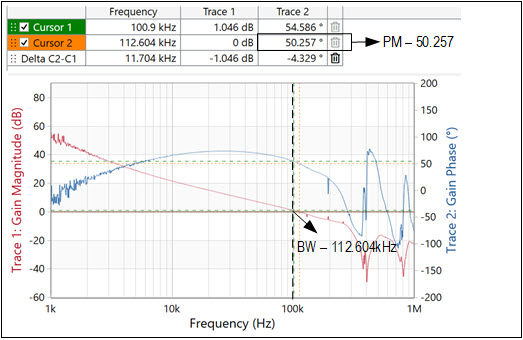The increment in the BW (frequency domain) is directly translated into improvement in the transient response (time domain) of the system, i.e., with an increased BW the system responds quicker to disturbances.

The response is tested using a pulsed load of 2A to 10A with 8A/µs ramp and the results are shown in Figure 9a and Figure 9b.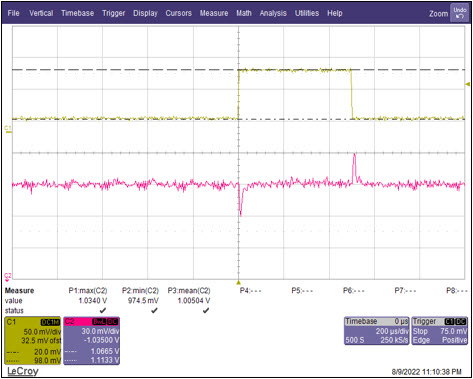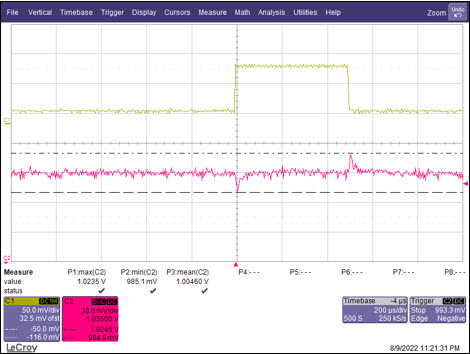Overshoot Uncompensated Overshoot in VOUT PEAK (mV) 28.8 Lead compensated Overshoot in VOUT PEAK (mV) 17.7 Δ VOUT PEAK - Overshoot reduction (mV) 11.1 Undershoot Uncompensated Undershoot in VOUT PEAK (mV) 30 Lead compensated Undershoot in VOUT PEAK (mV) 21.9 Δ VOUT PEAK - Undershoot reduction (mV) 8.1

It is observed that the undershoots and overshoots during the transient are reduced after improvement in BW by ~ 8mv to 10mV. Hence, this is a great way to meet the specification with a last-minute adjustment.

MAX20812 EV kit results for COUT = 870µF

Similar analysis is performed on the MAX20812 EV kit with the following component values shown in Table 4.

 RFB1 and RFB2 VOUT RLEAD (Ω) CLEAD (Calculated) CLEAD (Used in Ckt) fZ (kHz) fP (kHz) 3.01kΩ and 3.01kΩ 1V 0 25.6nF 25.3nF 2.09 4.18
 - BW (kHz) PM (°) No Load Uncompensated 41.341 64.924 Lead compensated 78.106 61.112 Full Load Uncompensated 46.893 68.28 Lead compensated 82.685 62.05

Again, from the Equation 7a, the maximum improvement achievable is 82.682kHz.

From results of Table 5, it is observed that the improvement exactly matches the calculated value of BWNEW, i.e., BWOLD scaled by the pole to zero frequency ratio.

The reason for more improvement with request to the MAX20710 is due to the difference in RFB1 and RFB2 values in the MAX20812 compared to the MAX20710 for the same output voltage, which ultimately changes the pole to zero frequency ratio in Equation 7a. Hence, for a good improvement in BW, the configuration with a lower value of RFB2 (compared to RFB1) is preferable.

As shown in Figure 10a and Figure 10b, the following are the bode plots for the loop gain for a Full load = 6A (values are shown in Table 5).The transient response improvement for a pulsed load of 1A to 6A with 5A/µs ramp is as follows: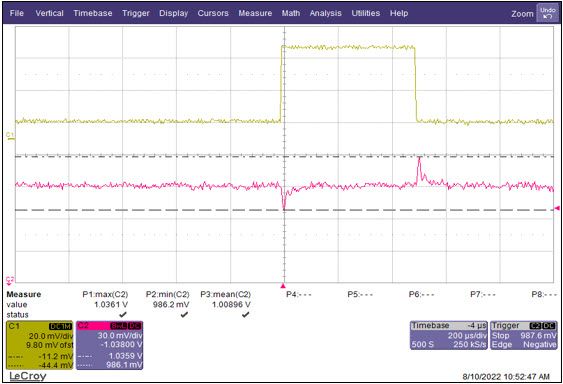Overshoot Uncompensated Overshoot in VOUT PEAK (mV) 48.1 Lead compensated Overshoot in VOUT PEAK (mV) 27.1 Δ VOUT PEAK - Overshoot reduction (mV) 21.1 Undershoot Uncompensated Undershoot in VOUT PEAK (mV) 33.5 Lead compensated Undershoot in VOUT PEAK (mV) 22.5 Δ VOUT PEAK - Undershoot reduction (mV) 11.1

The undershoot and overshoot in voltage is reduced by 10mV to 20mV, which is huge, and this is better compared to the previous case of the MAX20710. This is simply due to more increase in BW in the MAX20812 case.

From the above results, it is observed that lead compensation is achieved by the addition of a simple series RC network across RFB1, where the maximum improvement in BW is limited by the location of fZ and values of RFB1 and RFB2. This method of compensation is used to improve the BW only up to a certain extent; therefore, external compensation is used when all other components of the system are fixed with variable COUT requirements. Hence, if there is a requirement of last-minute BW modification (with/without PM improvement), the lead compensation is the best method to achieve it.

### Loop Gain with Lag Compensation

The main objective of lag compensation is to obtain improvement in phase margin (PM) and reduce the BW of the loop gain T(s) (assuming higher PM at lower BW for the system). This is done in cases where the system has a low COUT value, which causes the BW to near Switching frequency (fSW), leading to higher noise in the system.

This section provides a design method for component selection (RLAG and CLAG) with a goal of reducing the crossover and to obtain improvement in PM at the new BW.

Note: Do not maximize the BW reduction as this decreases the system performance.

Figure 12 shows a typical compensated current mode control system. With lag compensation included the overlay plot is the response.The design approach is slightly different from the lead compensation method. Here, the PM improvement is obtained from the system (i.e., without external compensation) and not from the lag compensation. In fact, lag compensation has a diminishing effect on the phase of the system as shown in Figure 4.

Therefore, for the lag compensated system, the criteria for the best improvement in PM (obtained from the system at a lower BW) is when:For calculation, the user can consider the boundary case, i.e.,Choose CLAG = 10nF (to obtain optimum BW reduction and avoid loop instability issues). Increasing the value of CLAG reduces the BW drastically as the fP is placed at frequencies less than 500Hz, which causes the gain plot to cross 0dB much faster.

The value of RLAG is calculated from the Result 2, given by:Equation 10

Hence, any value of RLAG greater than or equal to the value from Equation 10 provides the best result for the lag compensation.

For a lower BW, the fZ is shifted further down (i.e., fZ < 0.1BWOLD) by increasing the CLAG and reducing the value of RLAG. This shifts the pole-zero pair to lower frequencies which further improves the PM of the system.

The value of BWNEW obtained with lag compensation from the above RLAG and CLAG values provide the minimum reduction in fC so that the PM of the compensated system is not reduced due to lag compensation.

### Lag Compensation Hardware Testing and Results

The above theory is tested using both the MAX20710 and MAX20812 EV kits. For the test, a series R-C network is added in parallel with RFB2, with values for the series element calculated using the equations mentioned above. The components used for the experiment have tolerance of 1% (RLAG and CLAG). For both the cases, a CLAG of 10nF is used (as recommended).

Following are the results for each EV kits:

MAX20710 EV kit results with COUT = 800µF

Following are the values used for the test, it also includes the fZ and fP values obtained from the components used.

 RFB1 and RFB2 VOUT CLAG RLAG (Calculated) RLAG (Used in Ckt) fZ (kHz) fP (kHz) 1.87kΩ and 3.48kΩ 1V 10nF 1.35kΩ 1.5kΩ 12.566 6.41
 - BW (kHz) PM (°) No Load Uncompensated 125.669 37.984 Lag compensated 84.523 54.438 Full Load – 6A Uncompensated 127.192 38.13 Lag compensated 83.319 54.198

From the above results, it is observed that there is an improvement in PM by ~ 20° with reduction in the crossover frequency or BW. For slight modifications in the crossover, the RLAG is further adjusted (as explained previously), but this shifts the crossover only by a small margin.

Figure 13a and Figure 13b show the bode response of the loop gain with and without lag compensation at Full load = 10A (values are shown in Table 8).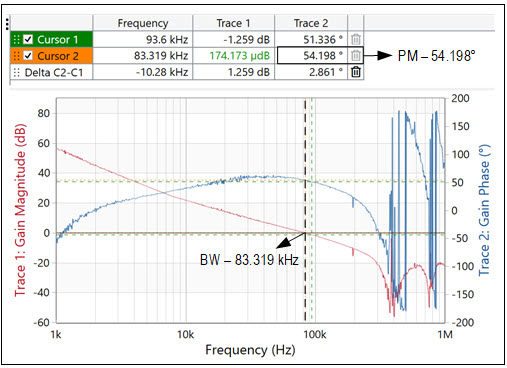MAX20812 EV kit results with COUT = 270.1µF

Similar analysis is performed on the MAX20812 EV kit with the following component values shown in Table 9.

 RFB1 and RFB2 VOUT CLAG RLAG (Calculated) RLAG (Used in Ckt) fZ (kHz) fP (kHz) 3.01kΩ and 3.01kΩ 1V 10nF 1.4249kΩ 1.42kΩ 11.169 6.025

Table 9 shows the components values and other calculated quantities.

 RFB1 and RFB2 VOUT CLAG RLAG (Calculated) RLAG (Used in Ckt) fZ (kHz) fP (kHz) 3.01kΩ and 3.01kΩ 1V 10nF 1.4249kΩ 1.42kΩ 11.169 6.025
 - BW (kHz) PM (°) No Load Uncompensated 111.69 55.714 Lag compensated 65.898 63.079 Full Load – 6A Uncompensated 131.651 52.574 Lag compensated 70.925 66.9

There is an improvement of ~11° in this case, which is lower than the previous case, as this improvement is from the system itself and not dependent on the compensation.

Figure 14a and Figure 14b show the bode plot of both the compensated and uncompensated system, at Full load = 6A (values are shown in Table 10).As mentioned, lag compensation is not used regularly, it is mainly used in cases where the system has low COUT count (i.e., high crossover frequency).

Although, through this method the user can push the BW to a much lower value, it is recommended not to go lower than 1/10 of fSW, as the system performance decreases mainly with respect to transient behavior.

Thereby, lag compensation is one of the best methods to improve the PM, with the added benefit of lowering the BW of the system.

### Conclusion

The results obtained for the external compensation hold true for the part numbers mentioned at the beginning of the document. It can also be applied to any other ADI's power management ICs which have the similar form of BW equation, i.e., proportional to the feedback ratio.

In such cases, it is advised to have a DNI/DNP pad for RC network across the feedback resistors, this brings in flexibility during the testing phase of the board to obtain the necessary stability margins.

All said, external compensation is one of the methods to boost the performance of the original system.

### 参考电路

1 SW Lee. (20014).Demystifying Type II and Type III Compensators Using Op-Amp and OTA for DC/DC Converters (Application Report No. SLVA662). Retrieved from Texas Instruments website: https://www.ti.com/lit/an/slva662/slva662.pdf.

2 R. W. Erickson and D. Maksimovic, Fundamentals of Power Electronics, 2nd ed. Norwell, MA, USA: Kluwer, 2001.